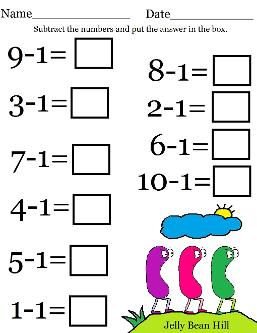Easter Math Subtraction Worksheet

This is a free printable Easter Math subtraction worksheet for kids to practice their subtraction. Have them subtract the numbers and put the answer in the box.

Easter- See All